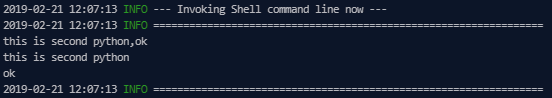An assignment node is a special node. It allows you to assign values to output parameters by writing code in the node and passes them with the node context to child nodes for reference.

Note You can only use assignment nodes in DataWorks Standard Edition or higher.

## Create an assignment node

1. Log on to the DataWorks console. In the left-side navigation pane, click Workspaces. On the Workspaces page, find the target workspace and click Data Analytics in the Actions column.
2. On the Data Analytics tab that appears, move the pointer over the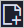icon and choose General > Assignment Node.

Alternatively, you can click a workflow in the left-side navigation pane, right-click General, and then choose Create > Assignment Node.

3. In the Create Node dialog box that appears, set Node Name and Location.
Note The node name must be 1 to 128 characters in length.
4. Click Commit.

## Write the value assignment logic

An assignment node has a fixed output parameter named outputs. You can view the information about this parameter in the Parameters section on the Properties tab. You can select ODPS SQL, SHELL, or Python from the Language drop-down list to write code and assign values to parameters. The value of the outputs parameter can be computed after the corresponding code is run. You can specify only one language for a single assignment node.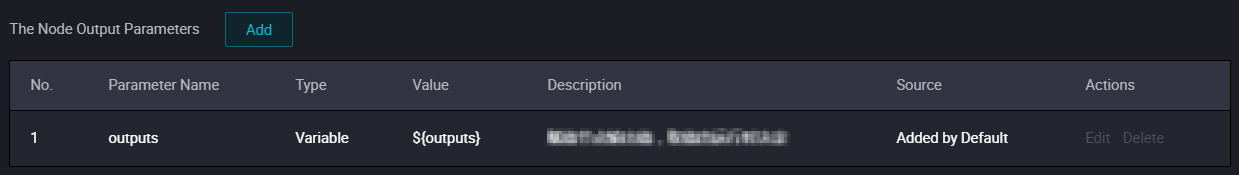The outputs parameter has the following limits:
• The value of the outputs parameter is taken only from the output of the last line of the code.
• For ODPS SQL, use the output of the SELECT statement in the last line.
• For SHELL, use the output of the ECHO statement in the last line.
• For Python, use the output of the PRINT statement in the last line.
• The passed value of the outputs parameter is limited to 2 MB in size. If the output of the assignment statement exceeds this limit, the assignment node fails to run.

## Use an assignment node as a parent node

After adding an assignment node as the parent node of a node, you can define the output of the assignment node as the input parameter of the child node in the node context. Then, reference the defined input parameter in the code of the child node. In this way, the child node can obtain the specific values from the output of the assignment node. For more information, see Context-based parameters.

## Example of the assignment node

2. Create two Shell nodes and four assignment nodes. On the workflow dashboard, configure the dependencies by drawing lines between nodes, as shown in the following figure.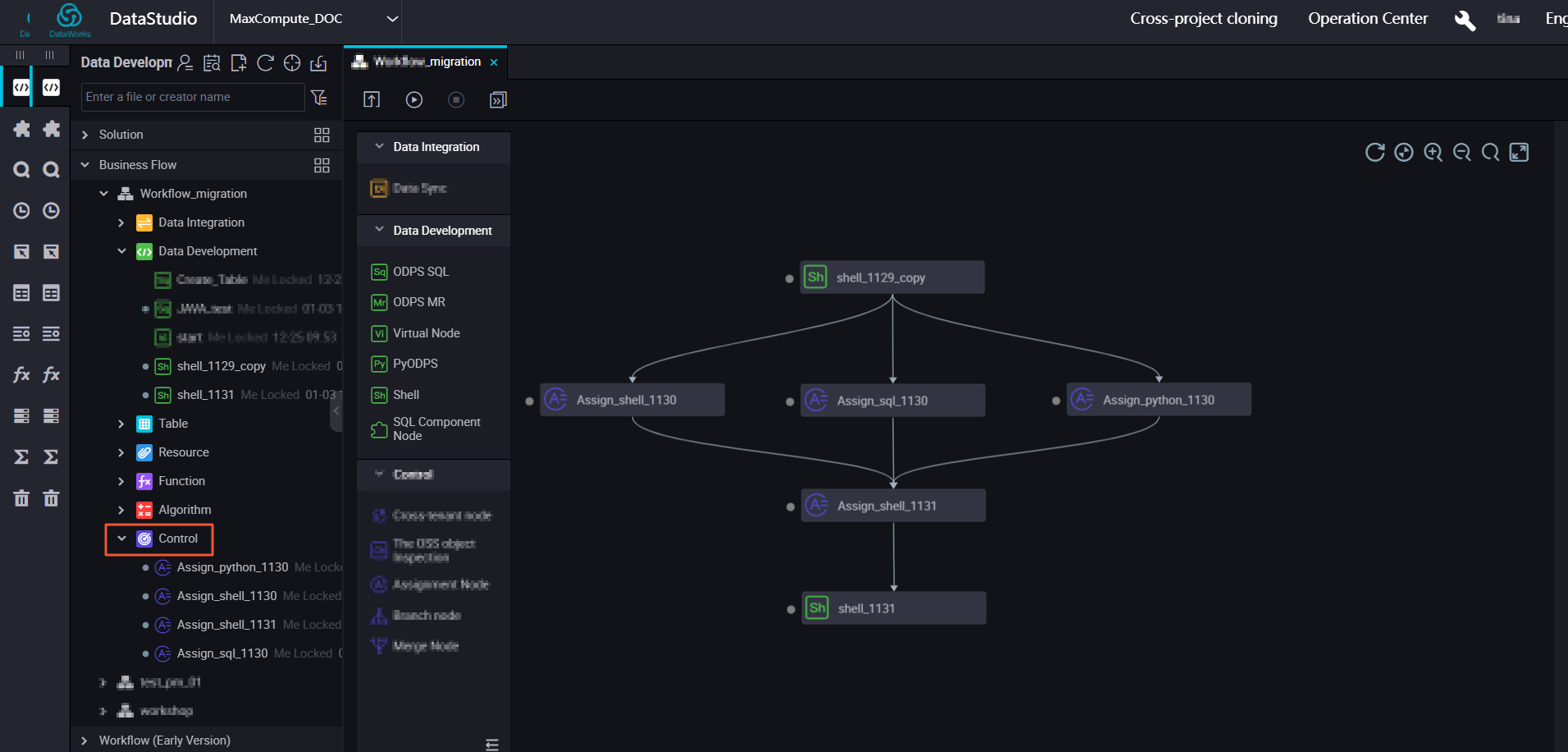3. When you configure an assignment node, the outputs parameter is automatically added by default. After running the assignment node, you can view the parameter value in the Parameters section on the Properties tab.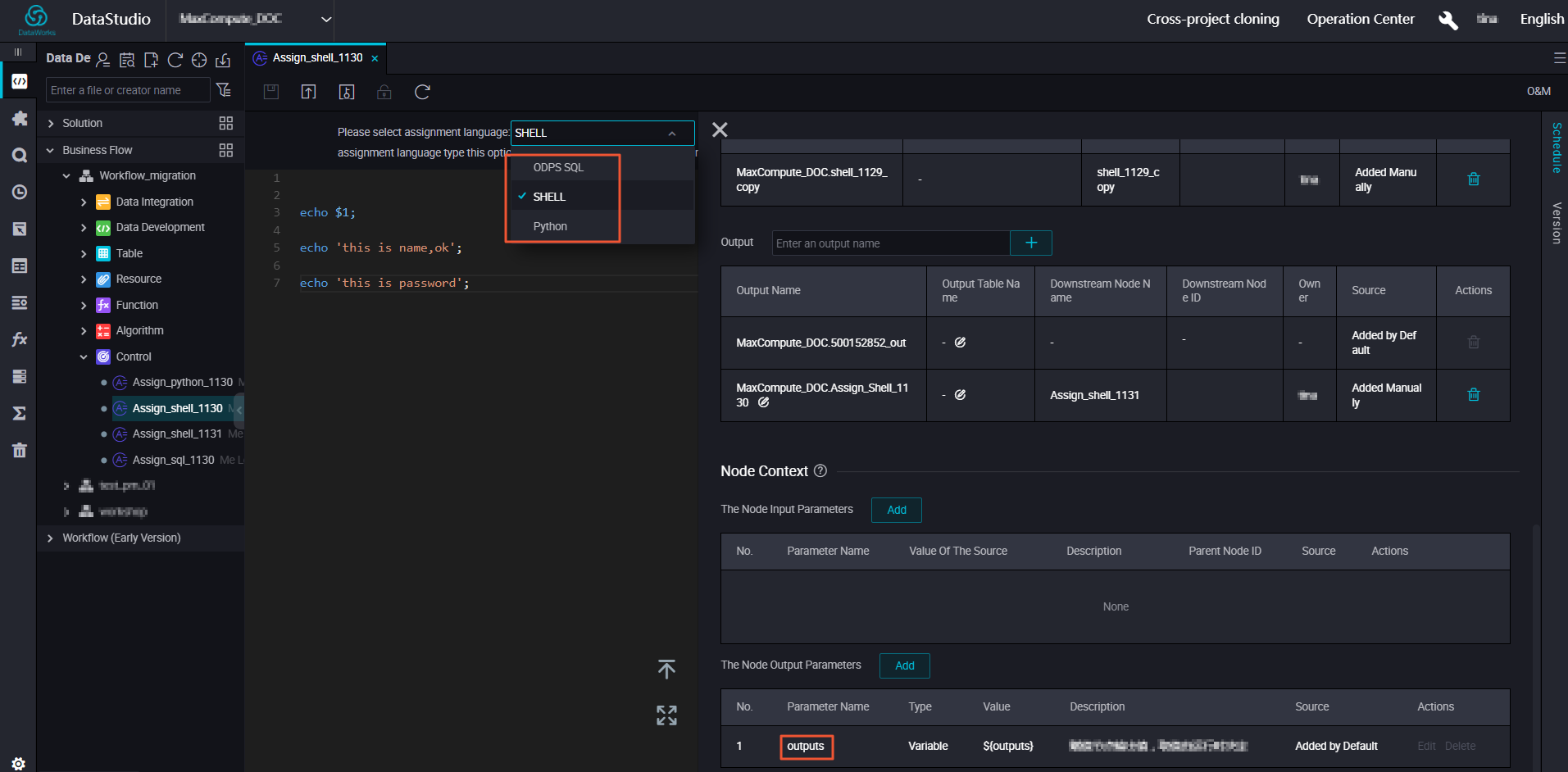4. Define the outputs parameter of the parent node as the input parameter of the child node.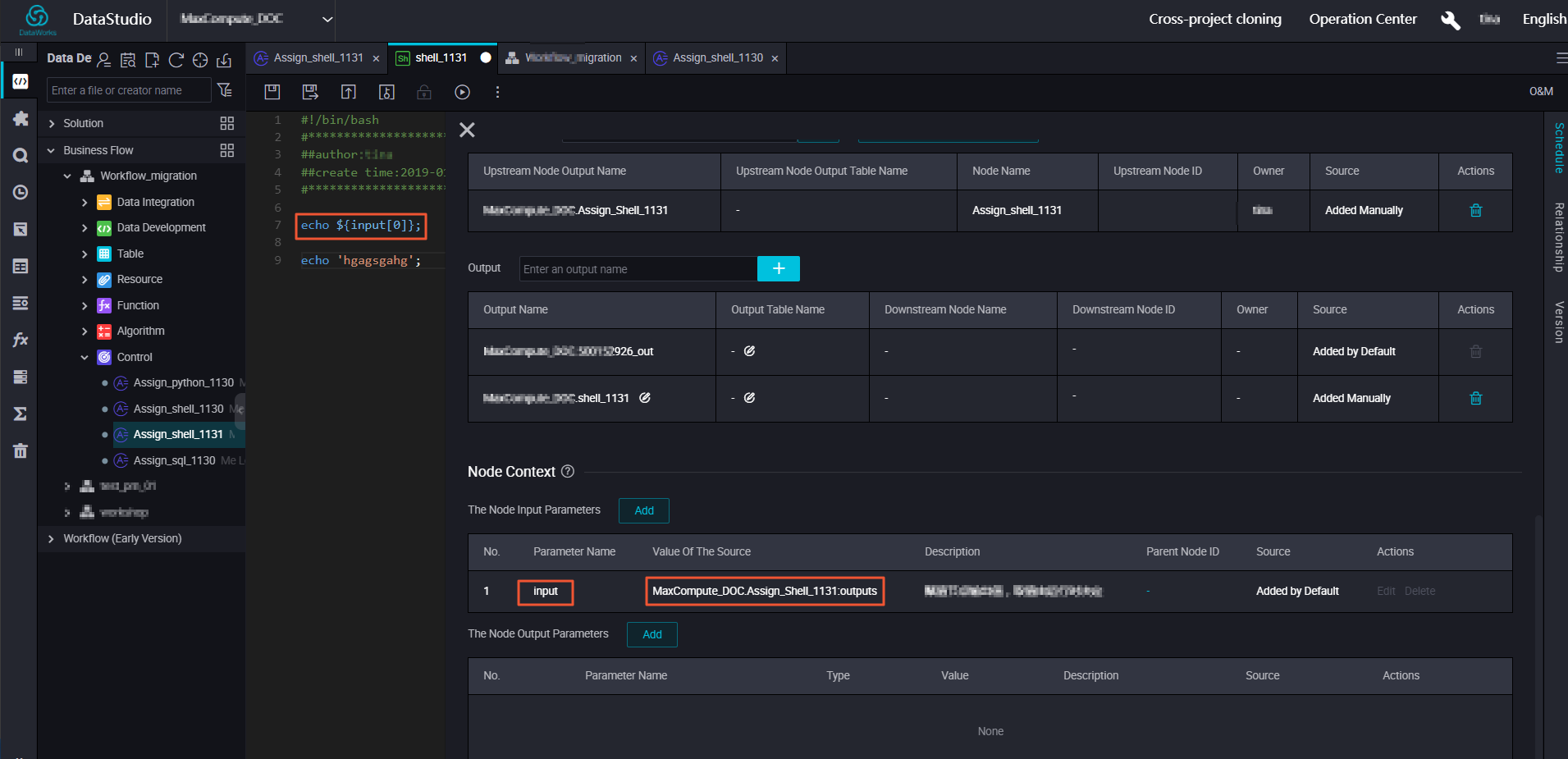## Run the assignment node

Note The preceding configurations can take effect through retroactive data generation in Operation Center, but do not take effect during testing.
1. After configuring the nodes in the workflow, commit the nodes for scheduling. Generally, node instances are generated and run on the next day.
2. When the instances are running, you can right-click a node in the directed acyclic graph (DAG) and select View Node Details to view the input and output parameters.
3. In the runtime logs, you can view the final output of the code in finalResult.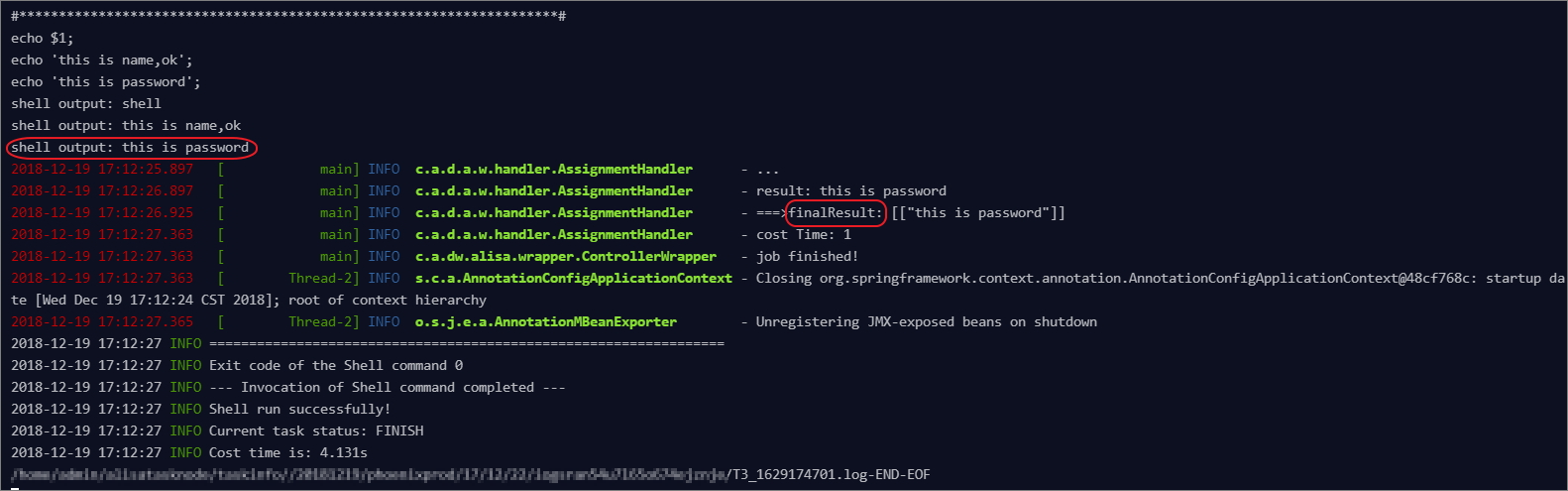## Summary

Note The value of the outputs parameter is the output of the last line of the code.
This section describes how to use ODPS SQL, SHELL, and Python to write code for an assignment node and define the arrays generated by the assignment node as the input parameter of a Shell node.
• ODPS SQL: The output of the assignment node is a one-dimensional or two-dimensional array.
In this example, the query result is a two-dimensional array.
``````2,this is name6
1,this is name5``````
The following figure shows the output code of the Shell node.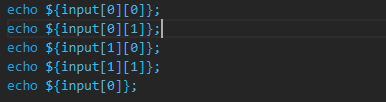The following figure shows the output of the Shell node.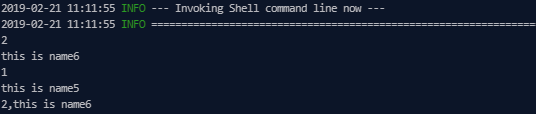• SHELL: The output of the assignment node is a one-dimensional array.
The following figure shows the output code of the Shell node.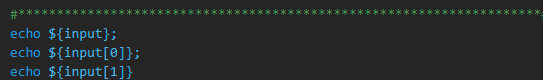The following figure shows the output of the Shell node.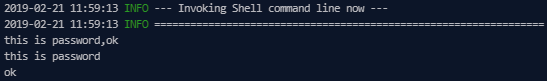• Python: The output of the assignment node is a one-dimensional array.
The following figure shows the output code of the Shell node.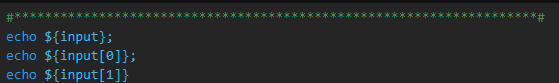The following figure shows the output of the Shell node.